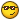## Recommended Posts

I'm currently writing a simple triangle solver app, but I'm having troubles when using the law of sines ( a/Sin(A) = b/Sin().

Here's my problem:

```#include <math.au3>
; When testing this on my calculator I get around 19 which is correct but this doesn't (-4.03)
MsgBox(0,"",(10/_Degree(Sin(10)))*_Degree(Sin(160)))
; Oh and neither does this:
MsgBox(0,"",(10/Sin(10))*Sin(160))
; This works however:
MsgBox(0,"",(10/_Degree(Sin(10)))*_Degree(Sin(10)))```

I know it's the stupid radians fault but how do I fix it?

Edited by monoceres

Broken link? PM me and I'll send you the file!

##### Share on other sites

When you use the Sin() function, you must always give it a paramater in radians, and it will always return a number in radians. I see at some points you are using 160 which is most likely degrees. Make sure you pay close attention to which numbers you use as paramaters.

##### Share on other sites

When you use the Sin() function, you must always give it a paramater in radians, and it will always return a number in radians. I see at some points you are using 160 which is most likely degrees. Make sure you pay close attention to which numbers you use as paramaters.

Of course how foolish of me.

Thanks for looking into itBroken link? PM me and I'll send you the file!

##### Share on other sites

Take a look at this:

#463161

##### Share on other sites

When you use the Sin() function, you must always give it a paramater in radians, and it will always return a number in radians. I see at some points you are using 160 which is most likely degrees. Make sure you pay close attention to which numbers you use as paramaters.

@Everyone, especially monoceres

This is not so. Sin(x) does not return a number in any particular unit. It merely returns the value of the ratio of the length of the opposite side to the length of the hypotenuse of one of the non right angles in a right triangle whose angle measure is x. The angle measure is traditionally given in radians for Sine, Cosine, and Tangent functions because radians are the natural units of a circle. The only reason we use degrees is because the greeks had some fascination with using degrees instead of the more natural radians.

In the world of the unit circle, Sin() is the value of the y coordinate on the unit circle while Cos() is the value of the x coordinate on the unit circle. Tan() is equal to the slope of the angle on the unit circle.

```ConsoleWrite(Round(Cos(_DegreesToRadians(90)),2)&@LF)

Local \$pi = 3.14159265358979
Return \$nDegrees * \$pi / 180 ; pi is equal to 180 degrees.  So remove the 180 and multiply the remaining value by pi(I did this backwards, but it doesn't matter).
EndFunc

Local \$pi = 3.14159265358979
Return \$nRadians * 180 / \$pi ; pi is equal to 180 degrees.  So remove the pi and multiply the remaining value by 180(I did this backwards, but it doesn't matter).
EndFuncoÝ÷ Ù¦zfÞ­× ­ç¬jwkiØÆ«z)éºÛ(~Ø^~éÜ¶*'J)Þ
"æ§u6§éíhºÚn¶Äáz­¦ëlj·­®((¶¸­«b¢Çè­ªâm®&§W¨­Ê(­Ø§j×¬¢»%¢¬¢v®+·%{br¯z¼(®Wr¢æ«{
+)àqÞ­è¬Ê«{¦¦WèqêÞ±«­¢+Ù5Í    ½à À°ÅÕ½ÐìÅÕ½Ðì° ÄÀ½M¥¸¡}ÉÍQ½I¥¹Ì ÄÀ¤¤¤©M¥¸¡}ÉÍQ½I¥¹Ì ÄØÀ¤¤¤()Õ¹}ÉÍQ½I¥¹Ì ÀÌØí¹ÉÌ¤(%1½°ÀÌØíÁ¤ôÌ¸ÄÐÄÔäÈØÔÌÔàäÜä(%IÑÕÉ¸ÀÌØí¹ÉÌ¨ÀÌØíÁ¤¼ÄàÀ)¹Õ¹```

- The Kandie Man ;-)

"So man has sown the wind and reaped the world. Perhaps in the next few hours there will no remembrance of the past and no hope for the future that might have been." & _"All the works of man will be consumed in the great fire after which he was created." & _"And if there is a future for man, insensitive as he is, proud and defiant in his pursuit of power, let him resolve to live it lovingly, for he knows well how to do so." & _"Then he may say once more, 'Truly the light is sweet, and what a pleasant thing it is for the eyes to see the sun.'" - The Day the Earth Caught Fire

##### Share on other sites

@Everyone, especially monoceres

This is not so. Sin(x) does not return a number in any particular unit. It merely returns the value of the ratio of the length of the opposite side to the length of the hypotenuse of one of the non right angles in a right triangle whose angle measure is x. The angle measure is traditionally given in radians for Sine, Cosine, and Tangent functions because radians are the natural units of a circle. The only reason we use degrees is because the greeks had some fascination with using degrees instead of the more natural radians.

In the world of the unit circle, Sin() is the value of the y coordinate on the unit circle while Cos() is the value of the x coordinate on the unit circle. Tan() is equal to the slope of the angle on the unit circle.

```ConsoleWrite(Round(Cos(_DegreesToRadians(90)),2)&@LF)

Local \$pi = 3.14159265358979
Return \$nDegrees * \$pi / 180 ; pi is equal to 180 degrees.  So remove the 180 and multiply the remaining value by pi(I did this backwards, but it doesn't matter).
EndFunc

Local \$pi = 3.14159265358979
Return \$nRadians * 180 / \$pi ; pi is equal to 180 degrees.  So remove the pi and multiply the remaining value by 180(I did this backwards, but it doesn't matter).
EndFuncoÝ÷ Ù¦zfÞ­× ­ç¬jwkiØÆ«z)éºÛ(~Ø^~éÜ¶*'J)Þ
"æ§u6§éíhºÚn¶Äáz­¦ëlj·­®((¶¸­«b¢Çè­ªâm®&§W¨­Ê(­Ø§j×¬¢»%¢¬¢v®+·%{br¯z¼(®Wr¢æ«{
+)àqÞ­è¬Ê«{¦¦WèqêÞ±«­¢+Ù5Í    ½à À°ÅÕ½ÐìÅÕ½Ðì° ÄÀ½M¥¸¡}ÉÍQ½I¥¹Ì ÄÀ¤¤¤©M¥¸¡}ÉÍQ½I¥¹Ì ÄØÀ¤¤¤()Õ¹}ÉÍQ½I¥¹Ì ÀÌØí¹ÉÌ¤(1½°ÀÌØíÁ¤ôÌ¸ÄÐÄÔäÈØÔÌÔàäÜä(IÑÕÉ¸ÀÌØí¹ÉÌ¨ÀÌØíÁ¤¼ÄàÀ)¹Õ¹```

- The Kandie Man ;-)

Thanks for the answer. I already solved it using the function _radian() which I guess does exactly like the same as your function.

Anyways, I'm almost done with my Triangle Solver, posting it laterBroken link? PM me and I'll send you the file!

##### Share on other sites

Thanks for the helpI've just posted my Triangle SolverBroken link? PM me and I'll send you the file!

## Create an account

Register a new account

• ### Recently Browsing   0 members

×

• Wiki

• Back

• #### Beta

• Git
• FAQ
• Our Picks
×
• Create New...